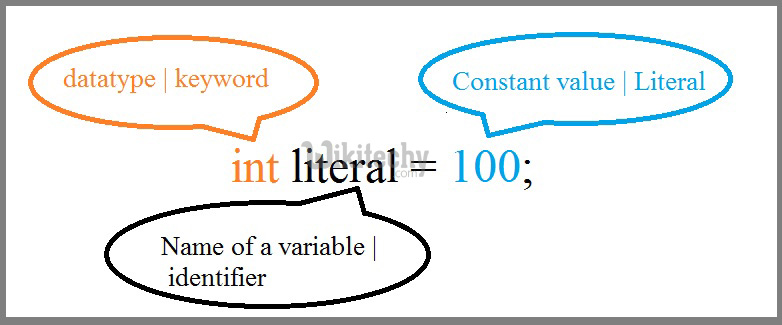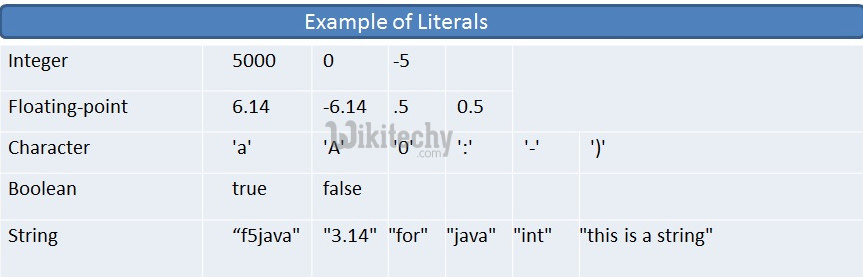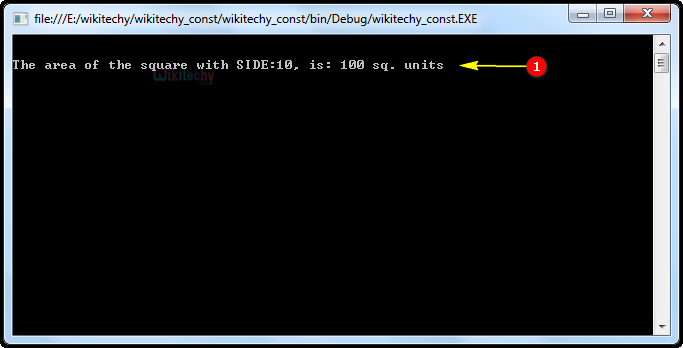# C# Const | C# Constants - c# - c# tutorial - c# netC# Const

## How to define constants in C# ?

• In constants, the const keyword to declare a constant field or a constant local.
• Constant fields and locals be located variables and may not be modified.
• Constants can be numbers, Boolean values, strings, or a null reference.
• Don’t generate a constant to represent information that we expect to change at any time.
• The constants are treated just like regular variables except that their values cannot be modified after their definition.## Types of Constant:

• Integer constants
• Floating-point constants
• Character Constants
• String constants

## Integer constants:

• An "integer constant" is a decimal (base 10), octal (base 8), or hexadecimal (base 16) number that represents an integral value.
• Integer constants are used to represent integer values that cannot be changed

## Example:## Floating-point constants

• A floating-point has an integer part, a decimal point, a fractional part, and an exponent part.

## Character Constants

• Character constant are enclosed in single quotes ( ‘ ’ ). For example, 'w' and it can be stored in a simple variable of char type.
 \\ \ character \' ' character \" " character \? ? character \n New line Formation \r Carriage return \f Form feed \a Alert or bell \b Backspace \v Vertical tab \t Horizontal tab \ooo Octal number of one to 3 digits \xhh . . . Hexadecimal number of one or more digits

## String constants:

• A string contains characters that are similar to character constants:
• Plain characters,
• Escape sequences, and
• Universal characters.

## Code Explanation1. In this example const int SIDE =10 is a constant integer data.
2. int area is specified as integer data type.
3. In this example, here we are using the variable name as area, and area=SIDE*SIDE its store the constant value of 10.
4. In this example, "The area of the square with SIDE" is represented as a console statement. SIDE is constant integer variable. area is specified as integer data type.

## Sample C# examples - Output :1. Here in this output "The area of the square with SIDE" is represented as a statement. And 10 is specifies the integer variable, 100 is specifies the area square of integer value 100.